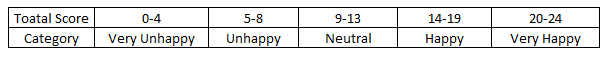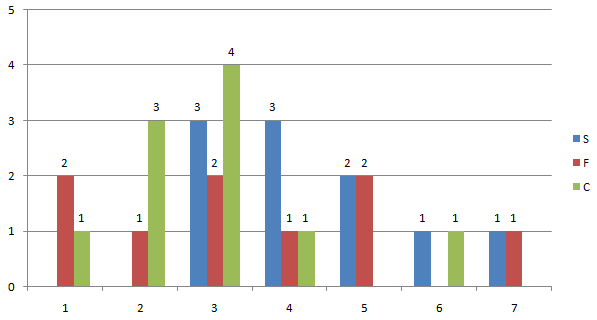# CAT 2017 LR questions

Simple Happiness index (SHI) of a country is computed on the basis of three, parameters: social support (S),freedom to life choices (F) and corruption perception (C). Each of these three parameters is measured on a scale of 0 to 8 (integers only). A country is then categorised based on the total score obtained by summing the scores of all the three parameters, as shown in the following table:Following diagram depicts the frequency distribution of the scores in S, F and C of 10 countries - Amda, Benga, Calla, Delma, Eppa, Varsa, Wanna, Xanda,Yanga and Zooma:Further, the following are known.

1. Amda and Calla jointly have the lowest total score, 7, with identical scores in all the three parameters.

2. Zooma has a total score of 17.

3. All the 3 countries, which are categorised as happy, have the highest score ln exactly one parameter.

Question: What is Amda's score in F?
Show Answer
Correct Answer: 1

Question: What is Zooma's score in S?
Show Answer
Correct Answer: 6

Question: Benga and Delma, two countries categorized as happy, are tied with the same total score. What is the maximum score they can have?
Options:
1. 14
2. 15
3. 16
4. 17
Show Answer
Correct Option: b

Question: If Benga scores 16 and Delma scores 15, then what is the maximum number of countries with a score of 13?
Options:
1. 0
2. 1
3. 2
4. 3
Show Answer
Correct Option: b